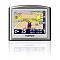## How To : Calculate The Distance Between Two Points

By David in How To. May 10, 2009

### How To

Add Phone Numbers to TomTom POIs
A quickie tip on adding phone numbers to tomtom's POIs

Convert Google My Maps KML Placemarks
Here's a useful little trick when using Google's My MapsCreate Your Own Points of Interest using POIEditor
New users to the site might want to read this guide on how to create your own Points of InterestInstall POIs to TomTom
Here's a beginners guide on how to install Points of Interest files directly on to your TomTom Navigator.

### General

Recent work
I've recently been working with the guys over at antiques selling site Antiques-Atla

Recently implemented a 'distance between UK post codes' system for a couple of web sites. Thought I'd share the PHP code for others that might be interested. It's using the Haversine formula to calculate the distance in kilometers between latitude and longitude points.

This it in action showing Leeds to London from Street Finder.co.uk

```\$A_lat = 53.1506;
\$A_lon = -1.8444;

\$B_lat = 52.2047;
\$B_lon = 0.1406;

\$source_lat =  \$A_lat * pi() / 180;

\$source_lon =  \$A_lon * pi() / 180;

\$dest_lat = \$B_lat * pi() / 180;

\$dest_lon = \$B_lon * pi() / 180;

\$R = 6371; // earth's mean radius in km

\$dLat  = \$dest_lat - \$source_lat;

\$dLong = \$dest_lon - \$source_lon;

\$a = sin(\$dLat/2) * sin(\$dLat/2) + cos(\$source_lat) *
cos(\$dest_lat) * sin(\$dLong/2) * sin(\$dLong/2);

\$b = 2 * atan2(sqrt(\$a), sqrt(1-\$a));

\$distance = \$R * \$b;
```